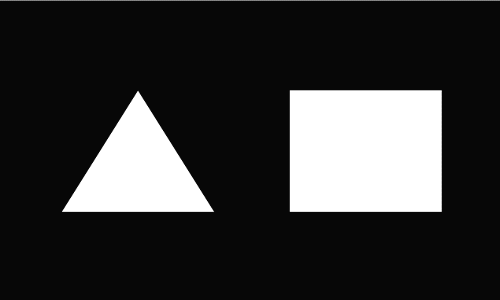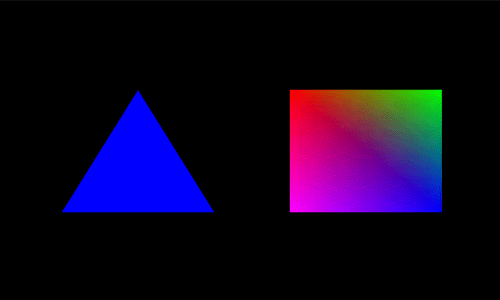# Programming - Games - OpenGL and C++

This toturial was written for me by Imran Khan (imranahmedkhan82@hotmail.com, iak1982@yahoo.com).
Copyright (c) 2004 is owned by Martin BakerDrawing Shapes:

In this Section we will draw some polygons on our OpenGL window ( which we have created in our previous tutorial ). We will just add some lines in our 'DrawGLScene' method.

 int DrawGLScene(GLvoid) { glClear(GL_COLOR_BUFFER_BIT | GL_DEPTH_BUFFER_BIT); glLoadIdentity(); glTranslatef(-1.5f,0.0f,-6.0f); glBegin(GL_TRIANGLES); glVertex3f( 0.0f, 1.0f, 0.0f); glVertex3f(-1.0f,-1.0f, 0.0f); glVertex3f( 1.0f,-1.0f, 0.0f); glEnd(); glTranslatef(3.0f,0.0f,0.0f); glBegin(GL_QUADS); glVertex3f(-1.0f, 1.0f, 0.0f); glVertex3f( 1.0f, 1.0f, 0.0f); glVertex3f( 1.0f,-1.0f, 0.0f); glVertex3f(-1.0f,-1.0f, 0.0f); glEnd(); return TRUE; }

First 2 lines are same. 3rd line calls a function 'glTranslatef(x, y, z)', which set the position where u want to draw the shap.Next we uses a method " glBegin " , it is use to draw points,triangle,quads etc. In this section we only draw Triangle & Quads. Other shapes which you can draw are :

GL_POINTS - dots
GL_LINES – lines, in pairs
GL_LINE_STRIP – polylines
GL_LINE_LOOP – closed loop
GL_TRIANGLES – triangles, three vertices
GL_POLYGON – convex filled polygon

The center of an OpenGL screen is 0.0f on the X and Y axis. To the left of center would be a negative number. To the right would be a positive number. Moving towards the top of the screen would be a positive number, moving to the bottom of the screen would be a negative number. Moving deeper into the screen is a negative number, moving towards the viewer would be a positive number.Here we choose to move left on x-axis (1.5f) , no movement in y-axis(0.0f) and 6.0f deeper ( z-axis). You can change to see wat effect it will produce on drawing.

Next we start drawing Triangle.glVertex3f got 3 parameters " x,y,z ". First ' glVertex3f ' is for top, Second ' glVertex3f ' is for bottom left and 3rd ' glVertex3f ' is for bottom right. Parameters of glVertex3f h (x,y,z ) has the same concept as we define for glTranslatef.

Next we start drawing a Sqaure. First ' glVertex3f ' is for top left, Second ' glVertex3f ' is for top right . 3rd ' glVertex3f ' is for bottom right. 4th ' glVertex3f ' is for bottom left.

Now when u run the program , you will get this output.We have drawn simple triangle and a sqaure. In this section we will add colors to it. We will fill triangle with a single color and fill sqaure with different shades of different colors.

The function we will call is ' glColor3f '. This function got 3 arguments ( red,Green,Blue ).You can create different colors by mixing up these values But the range of these values are from 0.0f to 1.0f. More the value more brighter will be the color.

We will add few lines in 'DrawGLScene' method.

 int DrawGLScene(GLvoid) { glClear(GL_COLOR_BUFFER_BIT | GL_DEPTH_BUFFER_BIT); glLoadIdentity(); glTranslatef(-1.5f,0.0f,-6.0f); glColor3f(0.0f,0.0f,1.0f); glBegin(GL_TRIANGLES); glVertex3f( 0.0f, 1.0f, 0.0f); glVertex3f(-1.0f,-1.0f, 0.0f); glVertex3f( 1.0f,-1.0f, 0.0f); glEnd(); glTranslatef(3.0f,0.0f,0.0f); glBegin(GL_QUADS); glColor3f(1.0f,0.0f,0.0f); //red glVertex3f(-1.0f, 1.0f, 0.0f); glColor3f(0.0f,1.0f,0.0f); //green glVertex3f( 1.0f, 1.0f, 0.0f); glColor3f(0.0f,0.0f,1.0f); //blue glVertex3f( 1.0f,-1.0f, 0.0f); glColor3f(1.0f,0.0f,1.0f); //pink glVertex3f(-1.0f,-1.0f, 0.0f); glEnd(); return TRUE; }

You see all the code is same we just add this line 'glColor3f(0.0f,0.0f,1.0f) ' before ' glBegin(GL_TRIANGLES)' . Actually we filling Triangle with Blue color.Thats why we write "1.0f" in the 3rd argument ( BLUE ) and remaining are Zero.

Next we fill Square with different shades of colors. You can see before every Vertex Draw , we define a color. i.e Every Vertex contain different color. First Vertex contain RED color , 2nd contain GREEN, 3rd contain BLUE and 4th vertex contain PINK color.In this way we can fill any shape with different colors.

When u run the program you will see this output.